## Statistics

### (NCERT Ex 14.1)Q1: A survey was conducted by a group of students as a part of their environment awareness programme, in which they collected the following data regarding the number of plants in 20 houses in a locality. Find the mean number of plants per house.

We use the following relation to find the class mark (xi) for each interval:

Class mark (xi) =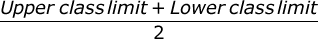Calculating xi and fixi as follows:

 Number of plants Number of houses(fi) xi fixi 0 -2 1 1 1 ☓ 1 = 1 2 -4 2 3 2 ☓ 3 = 6 4 -6 1 5 1 ☓ 5 = 5 6 -8 5 7 5 ☓ 7 = 35 8 - 10 6 9 6 ☓ 9 = 54 10 -12 2 11 2 ☓ 11 = 22 12 - 14 3 13 3 ☓ 13 = 39 Total 20 162

From the table, it is observed that

Σ fi = 20

Σ fixi = 162

Mean , x =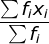== 8.1

Therefore, mean number of plants per house is 8.1.

Here, direct method has been used as the values of class marks (xi) and fi are small.

Q2: Consider the following distribution of daily wages of 50 worker of a factory.

 Daily wages(in Rs) 100 - 120 120 - 140 140 - 160 160 - 180 180 - 200 Number of workers 12 14 8 6 10

Find the mean daily wages of the workers of the factory by using an appropriate method.

To find the class mark (xi) for each interval, using the following relation:

Class mark (xi) =Class size (h) = 20

Taking 150 as assumed mean (a), di, ui, and fiui can be calculated as follows:

 Daily wages(in Rs) Number of workers(fi) xi di = xi - 150 ui =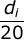fiui 100 - 120 12 110 -40 -2 -24 120 - 140 14 130 -20 -1 -14 140 - 160 8 150 0 0 0 160 - 180 6 170 20 1 6 180 - 200 10 190 40 2 20 Total 50 -12

Σ fi = 50

Σ fiui = -12

Mean , x = a +()h

= 150 + ()20

= 150 -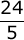= 150 - 4.8

= 145.2

∴ the mean daily wage of the workers of the factory = Rs 145.20.

Q3: The following distribution shows the daily pocket allowance of children of a locality. The mean pocket allowance is Rs.18. Find the missing frequency f.

 Daily pocket allownace(in Rs) 11 - 13 13 - 15 15 - 17 17 - 19 19 - 21 21 - 23 23 - 25 Number of workers 7 6 9 13 f 5 4

To find the class mark (xi) for each interval, using the following relation:

xi =Given that, mean pocket allowance, x = Rs 18

Taking 18 as assumed mean (a), di and fidi are calculated as:

 Daily pocket allowance(in Rs) Number of children(fi) Class mark(xi) di = xi - 18 fidi 11 - 13 7 12 -6 -42 13 - 15 6 14 -4 -24 15 - 17 9 16 -2 -18 17 - 19 13 18 0 0 19 - 21 f 20 2 2f 21 - 23 5 22 4 20 23 - 25 4 24 6 24 Total Σ fi = 44 + f 2f - 40

From the table:

Σ fi = 44 + f

Σ fidi = 2f - 40

x = a +18 = 18 + ()

0 =2f - 40 = 0

2f = 40

f = 20

∴ the missing frequency, f, is 20.

Q4: Thirty women were examined in a hospital by a doctor and the number of heart beats per minute were recorded and summarized as follows. Find the mean heart beats per minute for these women, choosing a suitable method.

 Number of heart beats per minute 65 - 68 68 - 71 71 - 74 74 - 77 77 - 80 80 - 83 83 - 86 Number of woman 2 4 3 8 7 4 2

To find the class mark (xi) for each interval, using the following relation:

xi =Class size, h, of this data = 3

Considering 75.5 as assumed mean (a), di, ui, fiui are calculated as follows.

 Number of heart beats per minute Number of woman(fi) xi di = xi - 75.5 ui =fiui 65 - 68 2 66.5 -9 -3 -6 68 - 71 4 69.5 -6 -2 -8 71 - 74 3 72.5 -3 -1 -3 74 - 77 8 75.5 0 0 0 77 - 80 7 78.5 3 1 7 80 - 83 4 81.5 6 2 8 83 - 86 2 84.5 9 3 6 Total 30 4

From the table:

Σ fi = 30

Σ fiui = 4

Mean , x = a +()☓ h

= 75.5 + 4/30 ☓ 3

= 75.5 + 0.4 = 75.9

∴ mean heart beats per minute for these women are 75.9 beats per minute.

Q5: In a retail market, fruit vendors were selling mangoes kept in packing boxes. These boxes contained varying number of mangoes. The following was the distribution of mangoes according to the number of boxes.

 Number of mangoes 50 - 52 53 - 55 56 - 58 59 - 61 62 - 64 Number of boxes 15 110 135 115 25

Find the mean number of mangoes kept in a packing box. Which method of finding the mean did you choose?

 Number of mangoes Number of boxes fi 50 - 52 15 53 - 55 110 56 - 58 135 59 - 61 115 62 - 64 25

Since the class intervals are not continuous, there is a gap of 1 between two class intervals. Therefore addingto the upper class limit and subtractfrom the lower class limit of each interval.

Obtaining Class mark (xi) by using the following relation.

xi =Class size (h) of this data = 3

Taking 57 as assumed mean (a), di, ui, fiuare calculated as:

 Class interval fi xi di = xi - 57 ui =fiui 49.5 - 52.5 15 51 -6 -2 -30 52.5 - 55.5 110 54 -3 -1 -110 55.5 - 58.5 135 57 0 0 0 58.5 - 61.5 115 60 3 1 115 61.5 - 64.5 25 63 6 2 50 Total 400 25

From the table:

Σ fi = 400

Σ fiui = 25

Mean , x = a +()☓ h

= 57 + ()☓ 3

= 57 + 3/16= 57 + 0.1875

= 57.1875

≈ 57.19

Mean number of mangoes kept in a packing box = 57.19.

Using step deviation method as the values of fi, di are big and also, there is a common multiple between all di.

Q6: The table below shows the daily expenditure on food of 25 households in a locality.

 Daily expenditure(in Rs) 100 - 150 150 - 200 200 - 250 250 - 300 300 - 350 Number of households 4 5 12 2 2

Find the mean daily expenditure on food by a suitable method.

To find the class mark (xi) for each interval, using the following relation:

xi =Class size = 50

Taking 225 as assumed mean (a), di, ui, fiui are calculated as:

 Daily expenditure(in Rs) fi xi di = xi - 225 ui =fiui 100 - 150 4 125 -100 -2 -8 150 - 200 5 175 -50 -1 -5 200 - 250 12 225 0 0 0 250 - 300 2 275 50 1 2 300 - 350 2 325 100 2 4 Total 25 -7

From the table:

Σ fi = 25

Σ fiui = -7

Mean , x = a +()☓ h

= 225 + (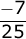)☓ 50

= 225 - 14

= 211

Thus the mean daily expenditure on food is Rs 211.

Q7: To find out the concentration of SO2 in the air (in parts per million, i.e., ppm), the data was collected for 30 localities in a certain city and is presented below:

 concentration of SO2 (in ppm) Frequency 0.00 - 0.04 4 0.04 - 0.08 9 0.08 - 0.12 9 0.12 - 0.16 2 0.16 - 0.20 4 0.20 - 0.24 2

To find the class mark (xi) for each interval, using the following relation:

xi =Class size of this data = 0.04

Taking 0.14 as assumed mean (a), calculating di, ui, fiui  as:

 concentration of SO2 (in ppm) Frequency(fi) Class mark(xi) di = xi - 0.14 ui =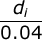fiui 0.00 - 0.04 4 0.02 -0.12 -3 -12 0.04 - 0.08 9 0.06 -0.08 -2 -18 0.08 - 0.12 9 0.10 -0.04 -1 -9 0.12 - 0.16 2 0.14 0 0 0 0.16 - 0.20 4 0.18 0.04 1 4 0.20 - 0.24 2 0.22 0.08 2 4

From the table:

Σ fi = 30

Σ fiui = -31

Mean , x = a +()☓ h

= 0.14 + ()☓ 0.04

= 0.14 - 0.04133

= 0.09867

≈ 0.099 ppm

Therefore, mean concentration of SO2 in the air = 0.099 ppm.

Q8: A class teacher has the following absentee record of 40 students of a class for the whole term. Find the mean number of days a student was absent.

 Number of days 0 - 6 6 - 10 10 - 14 14 - 20 20 - 28 28 - 38 38 - 40 Number of students 11 10 7 4 4 3 1

To find the class mark (xi) for each interval, using the following relation:

xi =Taking 17 as assumed mean (a), calculating di and fidi as:

 Number of days Number of students(fi) xi di = xi - 17 fidi 0 - 6 11 3 - 14 - 154 6 - 10 10 8 - 9 - 90 10 - 14 7 12 - 5 - 35 14 - 20 4 17 0 0 20 - 28 4 24 7 28 28 - 38 3 33 16 48 38 - 40 1 39 22 22 Total 40 - 181

From the table:

Σ fi = 40

Σ fidi = -181

Mean, x = a += 17 + ()

= 17 - 4.525

= 12.475

≈12.48

Therefore, the mean number of days = 12.48 days for which a student was absent.

Q9: The following table gives the literacy rate (in percentage) of 35 cities. Find the mean literacy rate.

 Literacy rate(in %) 45 - 55 55 - 65 65 - 75 75 - 85 85 - 95 Number of cities 3 10 11 8 3

To find the class mark (xi) for each interval, using the following relation:

xi =Class size(h) for this data = 10

Taking 70 as assumed mean (a), calculating di, ui, fiui :

 Literacy rate(in %) Number of cities(fi) xi di = xi - 70 ui =fiui 45 - 55 3 50 -20 -2 -6 55 - 65 10 60 -10 -1 -10 65 - 75 11 70 0 0 0 75 - 85 8 80 10 1 8 85 - 95 3 90 20 2 6 Total 35 -2

From the table:

Σ fi = 35

Σ fiui = -2

Mean , x = a +()☓ h

= 70 + ()☓ 10

= 70 -= 70 -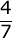= 70 - 0.57

= 69.43

Therefore, mean literacy rate is 69.43%.1.2.3.